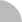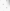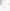# 臺灣博碩士論文加值系統

(3.236.110.106) 您好！臺灣時間：2021/07/25 08:38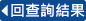:::

### 詳目顯示

: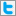Twitter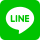•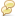被引用:0
•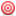點閱:221
•評分: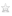•下載:11
•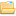書目收藏:0
 在一個無向圖 G=(V,E)之中，最密k集合為一個V的子集S，其點數為k，並且S所導出(induce)之子圖邊數為所有k集合中最多的，在社群網路中，最密k集合通常被認定為在此網路中關係密切的一群，最密k集合問題即使在最大分支度3的圖上，仍然為一個NP-Hard問題，在這篇論文中，我們定義了一個最密k集合問題的變化問題，並稱之為最密受限制k集合問題，當一個k集合導出的子圖之最小分支度為2時，我們稱之為受限制k集合，而最密受限制k集合導出之子圖邊數為所有受限制k集合中邊數最多的，我們在文中將證明如果我們有一個演算法可以在 O(f(n)) 時間複雜度之內解決最密受限制k集合問題，那我們將有一個複雜度為 O(poly(n)*f(n))的演算法解決最密k集合問題，我們也提出當我們的圖最大分支度為3，我們有一個時間複雜度為 O*(1.7485^n) 的演算法來解決最密受限制k集合問題。
 A densest k-set S in an undirected graph G=(V, E) is a vertex set of size k such that the number of edges in the subgraph induced by S is maximum among all subgraphs induced by k vertices. The concept of densest k-set is often used to define cohesive subgroups in a social network. The Densest k-Set problem is NP-hard even for graphs of maximum degree three. In this thesis, we define a variant problem of the Densest k-Set problem called the Densest Restricted k-Set problem. A vertex set S is said restricted if the graph induced by S has minimum degree at least two. The Densest Restricted k-Set problem is to find a densest k-set among all restricted vertex sets of size k. We show that if there is an algorithm that solves the Densest Restricted k-Set problem in O(f(n)) time, by taking the algorithm as a subroutine the Densest k-Set problem can be solved in time O(poly(n)*f(n)). Moreover, we give a polynomial-space algorithm running in time O*(1.7485^n) for the Densest Restricted k-Set problem on graphs of maximum degree~three.
 English AbstractContent ....................................1List of Tables .............................2List of Figures ............................3Introduction ...............................4Preliminaries ..............................10An O*(1.7485n)-time algorithm ..............14 The Densest Restricted k-set problem ....14 An O*(1.7485n)-time algorithm ....16Conclusion remarks .........................29Bibliography ...............................31
  S. Arora, D. Karger, and M. Karpinski, Polynimial time approximation schemes for dense instances of NP-hard problems, In Proceedings of the 27th Annual ACM Symposium on Theory of Computing, pp. 284–293,1995. R. Andersen and K. Chellapilla, Finding dense subgraphs with size bounds, In Proceedings of WAW'09, pp. 25–36, 2009. Y. Asahiro, K. Iwama, H. Tamaki and T. Tokuyama, Greedily finding a dense subgraph, Journal of Algorithms, 34 (2000), pp. 203–221. R.E. Bellman, Dynamic Programming, Princeton University Press, Princton, NJ, 1957. J. Backer and J. M. Keil, Constant factor approximation algorithms for the densest k-subgraph problem on proper interval graphs and bipartite permutation graphs, Information Processing Letters 110 (2010), pp. 635–638. A. Bhaskara, M. Charika, E. Chlamtac, U. Feige, and A. Vijayaraghavan, Detecting high log-densities: an O(n^(1/4))-approximation algorithms for the densest k-subgraph, In Proceedings of the 42nd ACM Symposium on Theory of Computing (STOC'10), pp. 201–210, 2010. A. Billonnet and F. Roupin, A deterministic approximation algorithm for the densest k-subgraph problem, Journal of Operational Research 3 (2008), pp. 301–314. N. Bourgeois, A. Giannakos, G. Lucarelli, I. Milis, V. Th. Paschos, Exact and approximation algorithms for densest k-subgraph, Technique report L. Cai. Parameterized complexity of cardinality constrained optimization problems. Comput. J., 51 (2008) 102–121. L. Cai, S. M. Chan, and S. O. Chan, Random separation: a new method for solving fixed-cardinality optimization problems, Proceedings of IWPEC 2006 LNCS 4169 (2006), pp. 239–250. M.-S. Chang, L.-H. Chen, L.-J. Hung, P. Rossmanith, and G.-H. Wu, Exact algorithms for problems related to the densest k-set problem, Proceedings of NCS 2011: Algorithms and Bioinformatics Workshop, pp. 1–9, 2011. D. Z. Chen, R. Fleischer, and Jian Li, Densest k-subgraph approximation on intersection graphs, In Proceedings of the 8th International Workshop on Approximation and Online Algorithms (WAOA'10), pp. 83–93, 2010. D. Corneil, Y. Perl, Clustering and domination in perfect graphs, Discrete Appl. Math. 9 (1984) 27–40. R. G. Downey and M. R. Fellows, Fixed-parameter tractability and completeness II: On completeness for W, Theor. Comput. Sci. 141 (1995) 109–131 K. Dudzinski, S. Walukiewicz, Exact methods for the knapsack problem and its generalizations, Eur. J. Oper. Res., 28 (1987), pp. 3–21. U. Feige and M. Seltser, On the dense k-subgraph problem, Technical Report, The Weizmann Institute, 1997. U. Feige, G. Kortsarz, and D. Peleg, The dense k-subgraph problem, Algorithmica 29 (2001), pp. 410–421. F. V. Fomin and D. Kratsch, Exact Exponential Algorithms, Springer, 2010. A. Goldberg, Finding a maximum density subgraph, Technical Report UCB/CSB 84/171, Department of Electrical Engineering and Computer Science, University of California, Berkeley, CA, 1984. J. Guo, R. Niedermeier, and S. Wernicke, Parameterized complexity of vertex cover variants, Theor. Comput. Syst. 41 (2007) 501–520. Q. Han, Y. Ye, and J. Zhang, An improved rounding method and semidefinite programming relaxation for graph partition, Mathematical Programming 92 (2002), pp. 509–535. R. Hassin, S. Rubinstein, and A. Tamir, Approximation algorithms for maximum dispersion, Operations Research Letters 21 (1997), pp. 133–137. J. M. Keil, and T. Brecht, The complexity of clustering in planar graphs, J. Combin. Math. Combin. Comput. 9 (1991) 155–159. S. Khot, Ruling out PTAS for graph min-bisection, dense k-subgraph, and bipartite clique, SIAM Journal on Computing 36 (2006), pp. 1025–1071. M. Liazi, I. Milis, and V. Zissimopoulos, The densest k-subgraph problem on clique graphs, J. Comb. Optim. 14 (2007) 465–474. M. Liazi, I. Milis, and V. Zissimopoulos, A constant approximation algorithm for the denest k-subgraph problem on chordal graphs, Information Processing Letters 108 (2008), pp. 29–32. T. Nonner, PTAS for densest k-subgraph in interval graphs, Manuscript, 2011. S. S. Ravi, D. J. Rosenkrantz, G. K. Tayi, Heuristic and special case algorithms for dispersion problems, Operations Research 42 (1994), pp. 299–310. A. Srivastav and K. Wolf, Finding dense subgraphs with semidefinite programming, In Proceedings of the International Workshop on Approximation Algorithms for Combinatorial Optimization, pp. 181–191, 1998.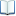電子全文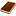國圖紙本論文推文當script無法執行時可按︰推文 網路書籤當script無法執行時可按︰網路書籤 推薦當script無法執行時可按︰推薦 評分當script無法執行時可按︰評分 引用網址當script無法執行時可按︰引用網址 轉寄當script無法執行時可按︰轉寄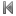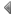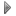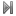top
 1 最大有限分支度一集合問題之啟發式演算法與分支化約演算法研究 2 分支化約演算法的複雜度分析：使用衡量與征服技巧

 1 廖述賢、張文榮(2010)。市場導向、創新能力、行銷能力與經營績效。商略學報，2(2)，87-107。 2 汪美伶、鄭雯憶(2007)。組織支持與服務導向組織公民行為－服務氣候之干擾角色。人力資源管理學報，7(4)，71-93。

 1 最大平衡子圖之演算法 2 最小連通支配集之演算法實作 3 最大配對導出子圖的正確解演算法 4 一些探測圖類別之辨識演算法設計 5 基於強健連結以偵測社會網路上之真實朋友 6 YouTube 影片分類統計瀏覽 7 基於區域確定性的群集設計主動學習樣本資料挑選的方法 8 街景清道夫:自Google街景圖中偵測與去除車輛 9 藉由改變最少正負號以平衡完全圖 10 Color CENTRIST：用於場景分類的顏色描述子 11 在有號完全圖上尋找大的平衡子集之演算法 12 基於信心指數找尋鄰近點進行離群點偵測 13 以不均勻比例及剪裁技術實作視訊縮放 14 以近似字詞比對方法輔助中醫症狀標準化 15 分支化約演算法的複雜度分析：使用衡量與征服技巧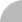簡易查詢 | 進階查詢 | 熱門排行 | 我的研究室